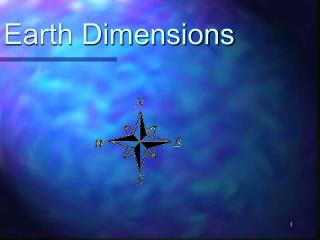DownloadDownload PresentationEarth Dimensions

# Earth Dimensions

Télécharger la présentation## Earth Dimensions

- - - - - - - - - - - - - - - - - - - - - - - - - - - E N D - - - - - - - - - - - - - - - - - - - - - - - - - - -
##### Presentation Transcript

1. Earth Dimensions

2. The Earth is an oblate spheroid. • That is a sphere with slightly flatten poles and a slight bulging at the equatorial region.

3. This means the polar circumference (40,008 km) is 68 km smaller than the equatorial circumference (40,076 km).

4. A. Evidence for the Earth shape comes from several sources.

5. 1. Early sailors noticed that ships seem to "disappear" as they sail out of port over the horizon.

6. However it is the altitude of Polaris that provides the earliest proof. • Polaris (the North Star) is directly overhead of the North Pole and has an altitude of 90⁰.

7. At the equator, the altitude of Polaris is 0⁰ (it is located on the horizon). • As you head north the altitude of Polaris increases, and is in fact equal to the latitude.

8. Finding Polaris Finding Polaris can be done using the "pointer" stars in the Big Dipper (Ursa Major). Facing Polaris, points you North, measuring the angle to Polaris tells you your latitude in the Northern Hemisphere

9. 2. Photographs from space confirm earth's oblate shape.

10. Careful measurements of a known mass shows that the mass' weight is heavier at the poles than at the equator.

11. B. How to determine the size of Earth? • Modern methods • Photographs from space • Measurements of Polaris (North Star) • Non-modern methods • Eratosthenes method

12. Eratosthenes • Greek mathematician • Lived approximately 2 x 103 years ago in Egypt • Used geometry to calculate the circumference of Earth

13. Assumptions Eratosthenes made • 1. All rays of the sun approach Earth in a parallel manner Rays of light sun Earth

14. Assumptions Eratosthenes made (cont.) • 2. Earth is a perfect sphere • He was almost correct

15. Geometry that Eratosthenes used Alternate interior angles made by parallel lines of the sun’s rays are equal sun a sun rays b angle a = angle b

16. sun A = Alexandria S = Syene Sun’s rays Distance between Alexandria and Syene was 925 km Shadow angles = 7.2° A S Earth’s center

17. How to find circumference? Use the information from the experiment to set up a proportion (2 equal ratios) Distance (km)Shadow Angle Circumference = 360° of Earth (km) 925 km cross multiply to solve 7.2° Circumference of Earth (km) = 360°

18. 925 km x 360° = circumference x 7.2° 925 km x 360° = circumference 7.2° 46, 250 km = circumference of Earth ( very close to the actual measurement)

19. C. The Outer Parts of the Earth.

20. Hydrosphere – the portion of the earth that contains earth's water. • This includes the (lower) atmosphere, surface, and upper layer of the lithosphere.

21. Atmosphere – the sphere of gases that surround the earth. The Atmosphere is layered, or divided into four zones based on temperature. The lowest, the troposphere contains most of the gases that we need to survive and our weather. The other three layers are the Stratosphere, Mesosphere, and Thermosphere. See Reference Table

22. Lithosphere (geosphere)– the outer most layer of the earth. Approximately 100 km thick, the lithosphere is the portion of the crust and mantle that contain the plates which move around forming earth's features.

23. Using a compass • A compass will not show True North (geographic north), but rather Magnetic North, because earth's magnetic field is tilted at 12⁰ angle to the Earth's axis of rotation